Successfully reported this slideshow.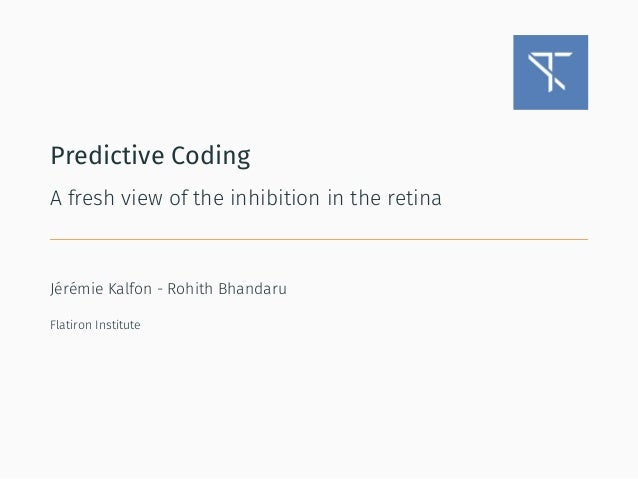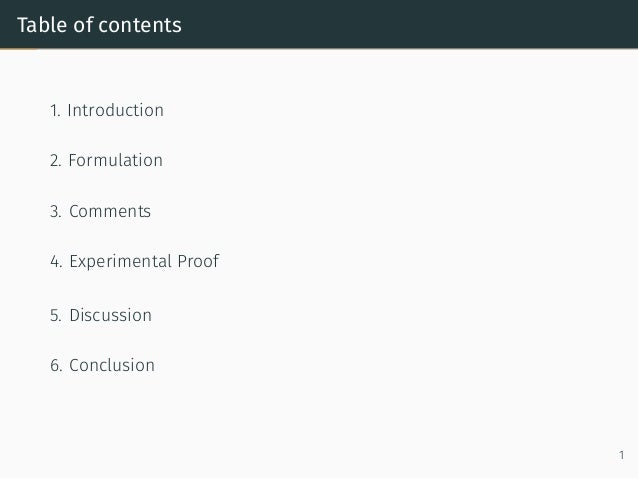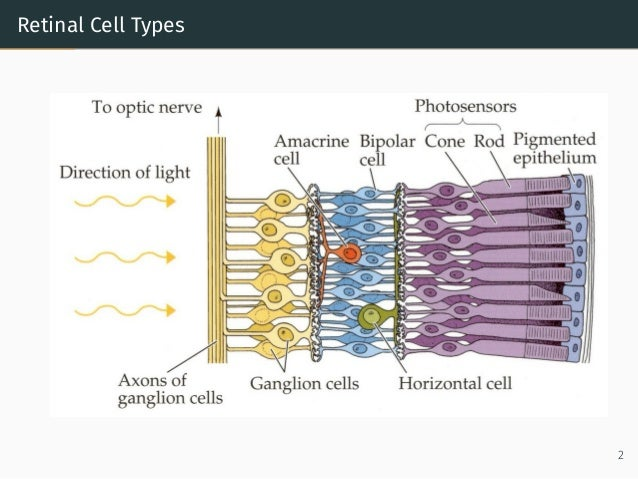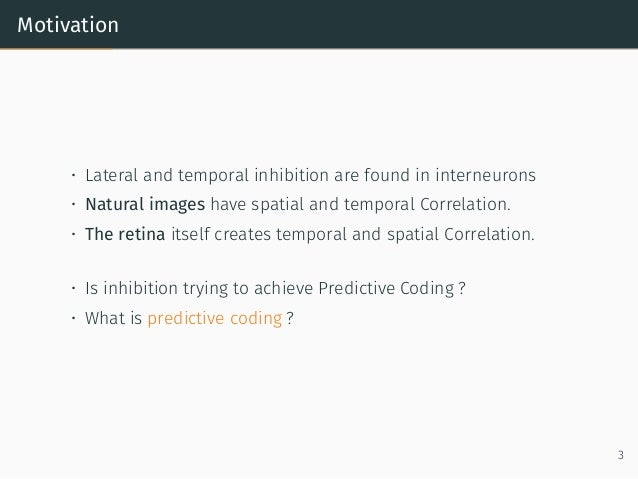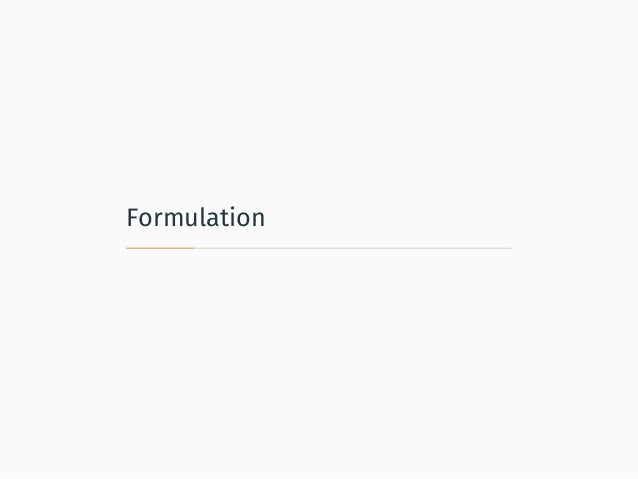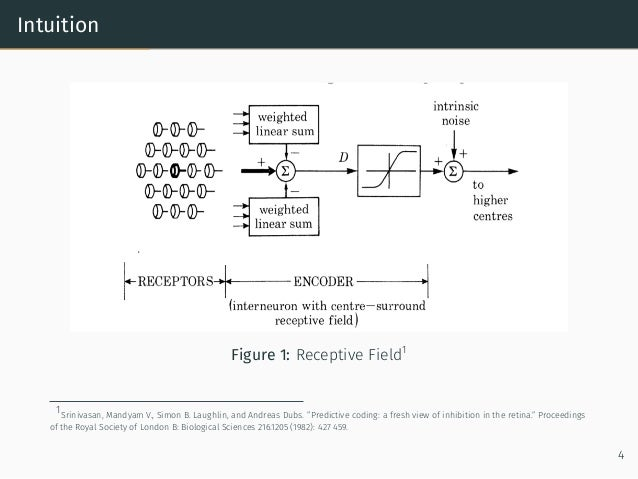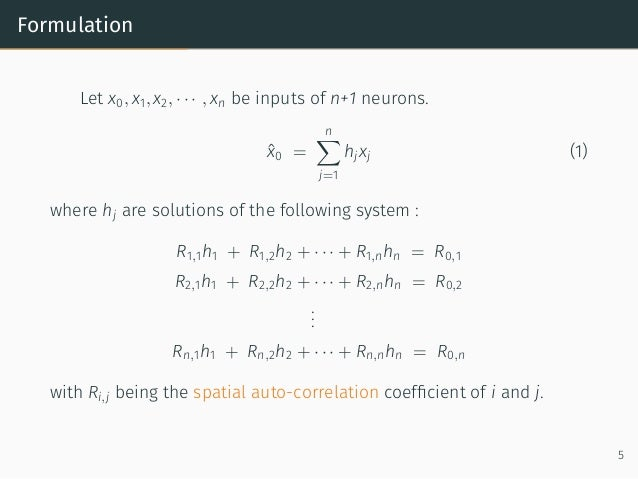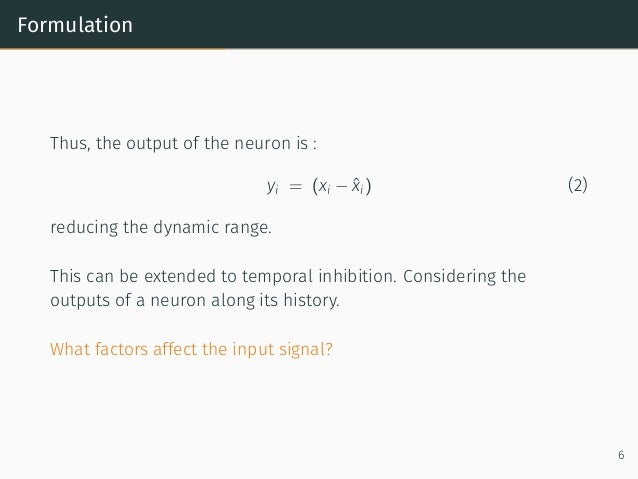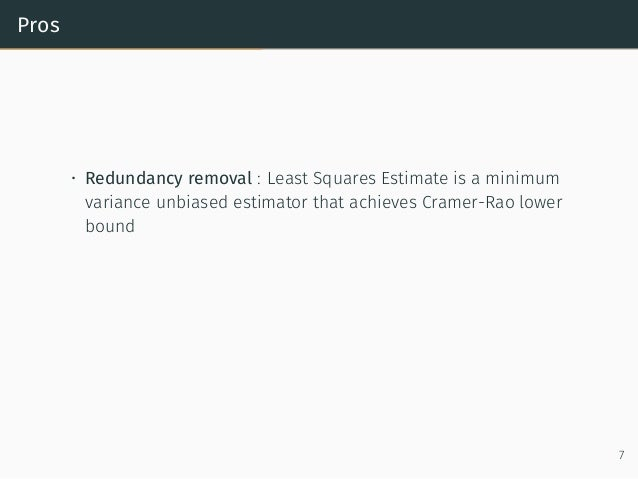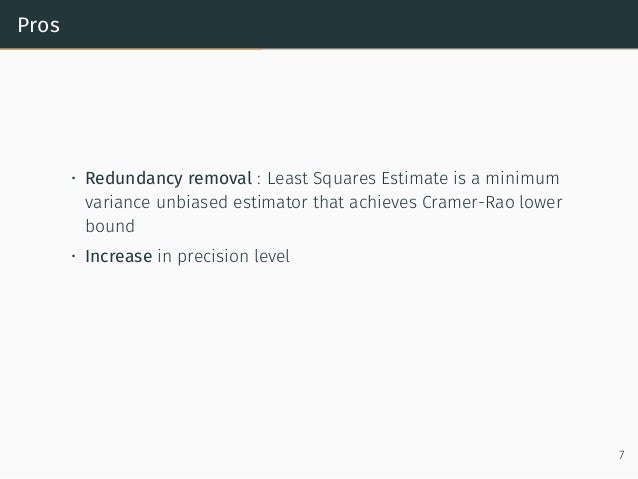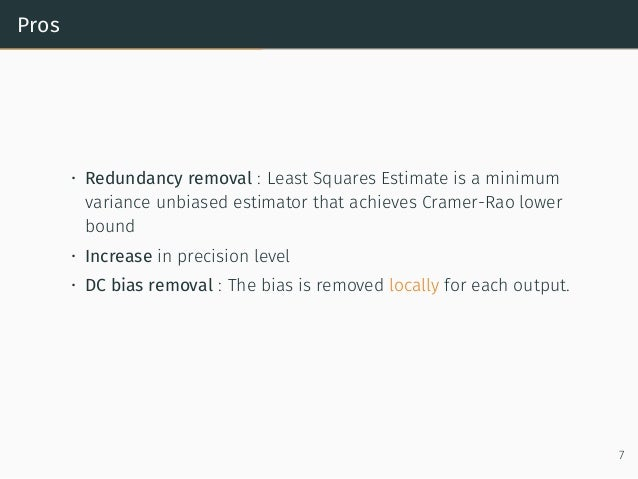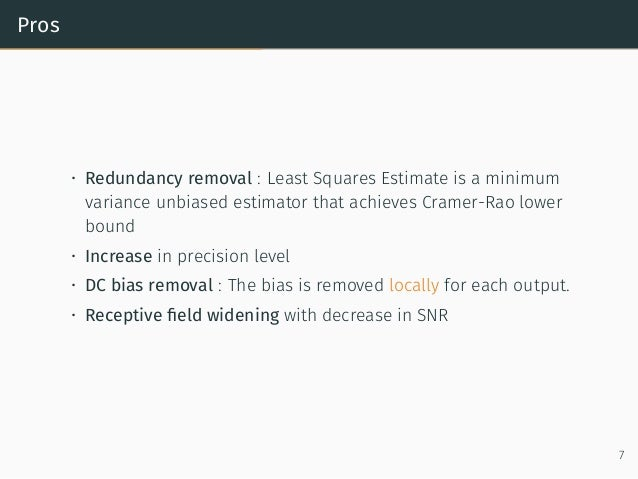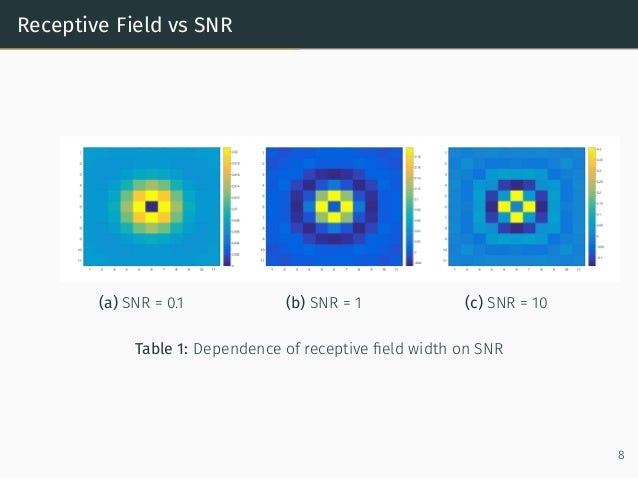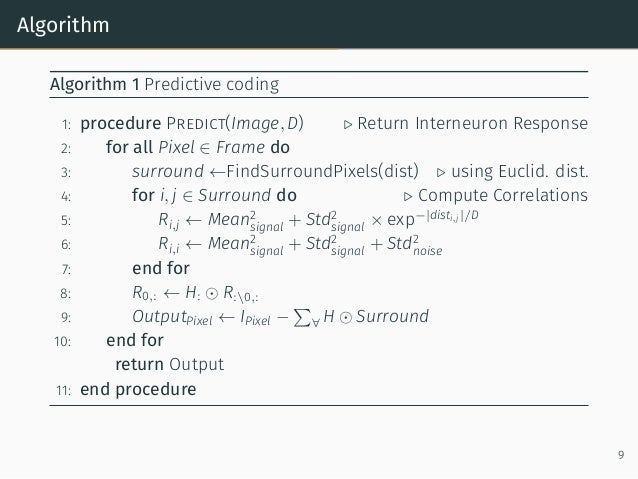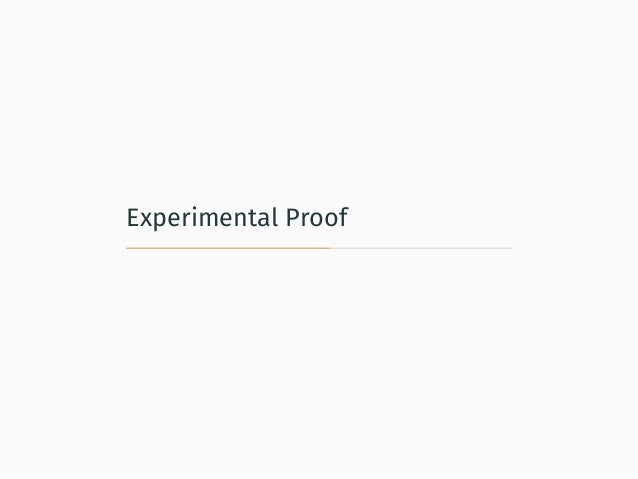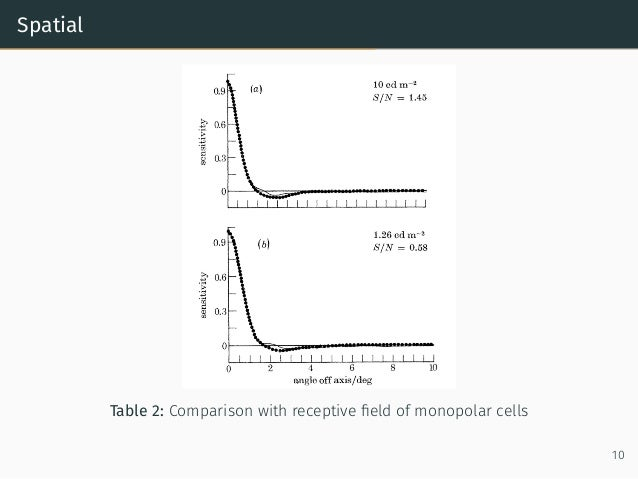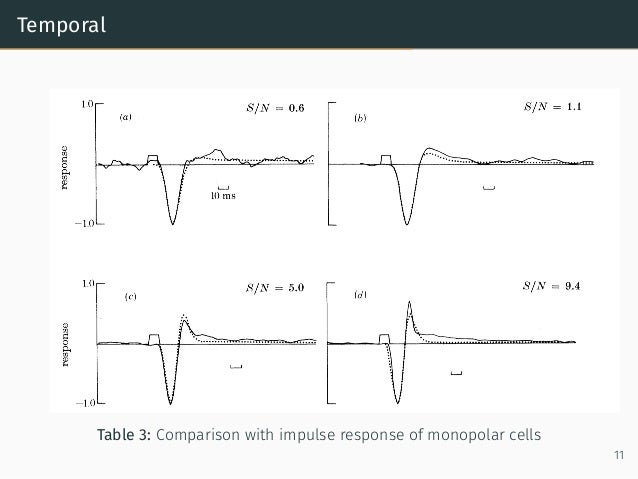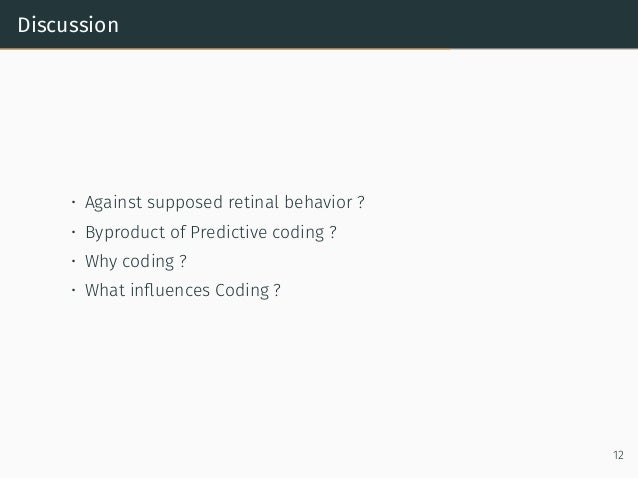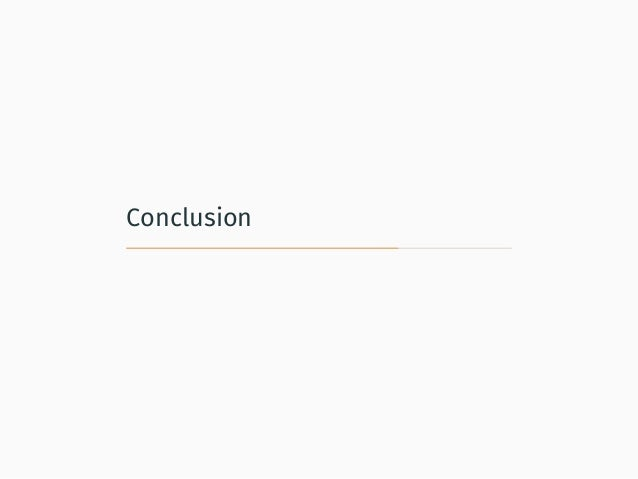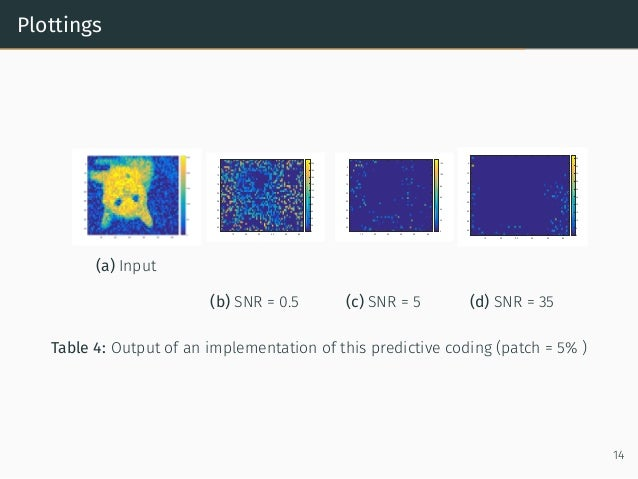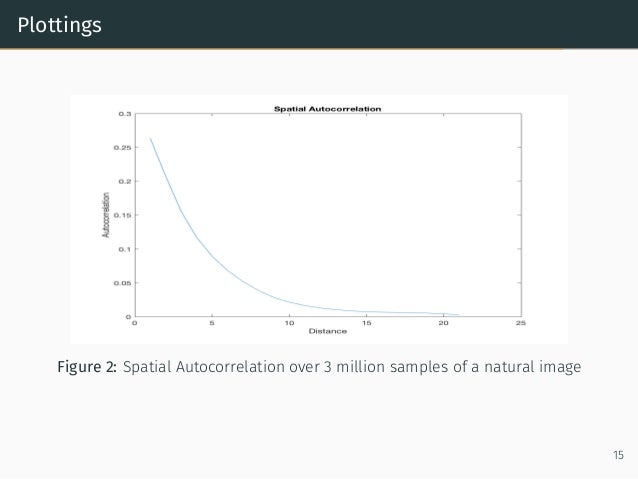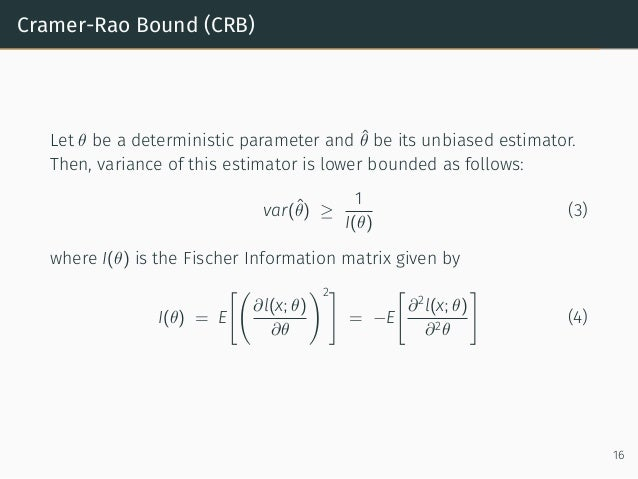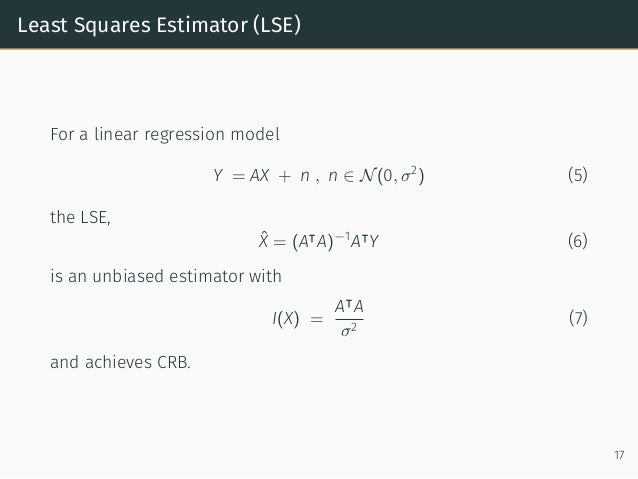Upcoming SlideShare
×
• Full Name
Comment goes here.

Are you sure you want to Yes No• Be the first to comment

• Be the first to like this

### Predictive coding : inhibition in the retina

1. 1. Predictive Coding A fresh view of the inhibition in the retina Jérémie Kalfon - Rohith Bhandaru Flatiron Institute
3. 3. Introduction
4. 4. Retinal Cell Types 2
5. 5. Motivation • Lateral and temporal inhibition are found in interneurons • Natural images have spatial and temporal Correlation. • The retina itself creates temporal and spatial Correlation. • Is inhibition trying to achieve Predictive Coding ? • What is predictive coding ? 3
6. 6. Formulation
7. 7. Intuition Figure 1: Receptive Field1 1Srinivasan, Mandyam V., Simon B. Laughlin, and Andreas Dubs. ”Predictive coding: a fresh view of inhibition in the retina.” Proceedings of the Royal Society of London B: Biological Sciences 216.1205 (1982): 427 459. 4
8. 8. Formulation Let x0, x1, x2, · · · , xn be inputs of n+1 neurons. ˆx0 = n∑ j=1 hjxj (1) where hj are solutions of the following system : R1,1h1 + R1,2h2 + · · · + R1,nhn = R0,1 R2,1h1 + R2,2h2 + · · · + R2,nhn = R0,2 ... Rn,1h1 + Rn,2h2 + · · · + Rn,nhn = R0,n with Ri,j being the spatial auto-correlation coefﬁcient of i and j. 5
9. 9. Formulation Thus, the output of the neuron is : yi = (xi − ˆxi) (2) reducing the dynamic range. This can be extended to temporal inhibition. Considering the outputs of a neuron along its history. What factors affect the input signal? 6
11. 11. Pros • Redundancy removal : Least Squares Estimate is a minimum variance unbiased estimator that achieves Cramer-Rao lower bound 7
12. 12. Pros • Redundancy removal : Least Squares Estimate is a minimum variance unbiased estimator that achieves Cramer-Rao lower bound • Increase in precision level 7
13. 13. Pros • Redundancy removal : Least Squares Estimate is a minimum variance unbiased estimator that achieves Cramer-Rao lower bound • Increase in precision level • DC bias removal : The bias is removed locally for each output. 7
14. 14. Pros • Redundancy removal : Least Squares Estimate is a minimum variance unbiased estimator that achieves Cramer-Rao lower bound • Increase in precision level • DC bias removal : The bias is removed locally for each output. • Receptive ﬁeld widening with decrease in SNR 7
15. 15. Receptive Field vs SNR (a) SNR = 0.1 (b) SNR = 1 (c) SNR = 10 Table 1: Dependence of receptive ﬁeld width on SNR 8
16. 16. Algorithm Algorithm 1 Predictive coding 1: procedure Predict(Image, D) ▷ Return Interneuron Response 2: for all Pixel ∈ Frame do 3: surround ←FindSurroundPixels(dist) ▷ using Euclid. dist. 4: for i, j ∈ Surround do ▷ Compute Correlations 5: Ri,j ← Mean2 signal + Std2 signal × exp−|disti,j|/D 6: Ri,i ← Mean2 signal + Std2 signal + Std2 noise 7: end for 8: R0,: ← H: ⊙ R:0,: 9: OutputPixel ← IPixel − ∑ ∀ H ⊙ Surround 10: end for return Output 11: end procedure 9
17. 17. Experimental Proof
18. 18. Spatial Table 2: Comparison with receptive ﬁeld of monopolar cells 10
19. 19. Temporal Table 3: Comparison with impulse response of monopolar cells 11
20. 20. Discussion
21. 21. Discussion • Against supposed retinal behavior ? • Byproduct of Predictive coding ? • Why coding ? • What inﬂuences Coding ? 12
22. 22. Conclusion
23. 23. Summary Thanks to the Simons Foundation and our Mentors github.com/jkobject/predictivecoding cba 13
24. 24. Plottings (a) Input 10 20 30 40 50 60 5 10 15 20 25 30 35 40 0 20 40 60 80 100 120 140 160 180 200 (b) SNR = 0.5 10 20 30 40 50 60 5 10 15 20 25 30 35 40 0 20 40 60 80 100 120 (c) SNR = 5 10 20 30 40 50 60 5 10 15 20 25 30 35 40 0 5 10 15 20 25 30 35 40 45 50 (d) SNR = 35 Table 4: Output of an implementation of this predictive coding (patch = 5% ) 14
25. 25. Plottings Figure 2: Spatial Autocorrelation over 3 million samples of a natural image 15
26. 26. Questions? 15
27. 27. Cramer-Rao Bound (CRB) Let θ be a deterministic parameter and ˆθ be its unbiased estimator. Then, variance of this estimator is lower bounded as follows: var(ˆθ) ≥ 1 I(θ) (3) where I(θ) is the Fischer Information matrix given by I(θ) = E [( ∂l(x; θ) ∂θ )2] = −E [ ∂2 l(x; θ) ∂2θ ] (4) 16
28. 28. Least Squares Estimator (LSE) For a linear regression model Y = AX + n , n ∈ N(0, σ2 ) (5) the LSE, ˆX = (A⊺ A)−1 A⊺ Y (6) is an unbiased estimator with I(X) = A⊺ A σ2 (7) and achieves CRB. 17

Total views

236

On Slideshare

0

From embeds

0

Number of embeds

0

2

Shares

0